Questions and exercises for Review

1.           What steps should one take when solving a problem using a computer?

2.             Explain some issues when dealing with the representation of real world objects in a computer program.

3.             Explain the notions: model of computation; computational problem; problem instance; algorithm; and program

4.             Show the algorithm design algorithm.

5.             What might be the resources considered in algorithm analysis?

6.             Explain the big-oh class of growth.

7.             Explain the big-omega class of growth.

8.             Explain the big-theta class of growth.

9.             What are the steps in mathematical analysis of nonrecursive algorithms?

10.        What are the steps in mathematical analysis of recursive algorithms?

11.        From lowest to highest, what is the correct order of the complexities O(n2), O(3n), O(2n), O(n2 lg n), O(1), O(n lg n), O(n3), O(n!), O(lg n), O(n)?

12.        What are the complexities of T1(n) = 3n lg n + lg n; T2(n) = 2n + n3 + 25; and T3(n, k) = k + n, where k less-than or equal to n? From lowest to highest, what is the correct order of the resulting complexities?

13.        Suppose we have written a procedure to add m square matrices of size n × n. If adding two square matrices requires O (n2 ) running time, what is the complexity of this procedure in terms of m and n?

14.        Suppose we have two algorithms to solve the same problem. One runs in time T1(n) = 400n, whereas the other runs in time T2(n) = n2. What are the complexities of these two algorithms? For what values of n might we consider using the algorithm with the higher complexity?

15.        How do we account for calls such as memcpy and malloc in analyzing real code? Although these calls often depend on the size of the data processed by an algorithm, they are really more of an implementation detail than part of an algorithm itself.

16.        Explain the stack ADT.

17.        Explain the list ADT.

18.        There are occasions when arrays have advantages over linked lists. When are arrays preferable?

19.        Explain the queue ADT.

20.        Sometimes we need to remove an element from a queue out of sequence (i.e., from somewhere other than the head). What would be the sequence of queue operations to do this if in a queue of five requests, req1, . . . , req5, we wish to process req1, req3 , and req5 immediately while leaving req2 and req4 in the queue in order? What would be the sequence of linked list operations to do this if we morph the queue into a linked list?

21.        Recall that each of the linked list data structures presented at the laboratory has a size member. The SLList and DLList data structures also contain a first and last member. Why are each of these members included?

22.        When would you use a doubly-linked list instead of a singly-linked one? Why?

23.        Show the result of inserting the numbers 32, 11, 22, 15, 17, 2, -3 în a doubly linked list with a sentinel.

24.        Show the result of inserting the numbers 32, 11, 22, 15, 17, 2, -3 în a circular queue of capacity 9.

25.        Show the result of inserting the numbers 32, 11, 22, 15, 17, 2, -3 în a stack of capacity 12.

26.        Determine the value returned by the function (depending on n), and the worst case running time, in big-Oh notation, of the following program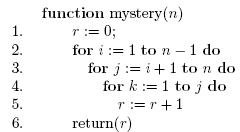27.        Determine the value returned by the function (depending on n), and the worst case running time, in big-Oh notation, of the following program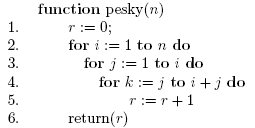28.        Define the term "rooted tree" both formally and informally.

29.        Define the terms ancestor, descendant, parent, child, sibling as used with rooted trees.

30.        Define the terms path, height, depth, level as used with rooted trees.

31.        Show the preorder traversal of the tree given in Fig. 1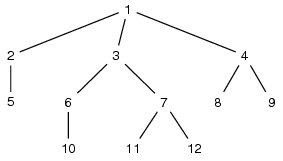Fig. 1. Example Tree

23.        Show the postorder traversal of the tree given in Fig. 1

24.        Show the inorder traversal of the tree given in Fig. 1

25.        Construct the tree whose preorder traversal is: 1, 2, 5, 3, 6, 10, 7, 11, 12, 4, 8, 9, and inoder traversal is 5, 2, 1, 10, 6, 3, 11, 7, 12, 8, 4, 9.

26.        Construct the tree whose postorder traversal is: 5, 2, 10, 6, 11, 12, 7, 3, 8, 9, 4, 1, and inoder traversal is 5, 2, 1, 10, 6, 3, 11, 7, 12, 8, 4, 9.

27.        Show the vector contents for an implementation of the tree in Fig. 1.

28.        Show the contents of the data structures (in a sketch) for an implementation of the tree in Fig. 1 using lists of children.

29.        Show the contents of the data structures (in a sketch) for an implementation of the tree in Fig. 1 using leftmost child - right sibling method.

30.        Show the binary search tree which results after inserting the nodes with keys 5, 2, 10, 6, 11, 12, 7, 3, 8, 9, 4, 1 in that order, in an empty tree.

31.        Show the binary search tree resulted after deleting keys 10, 5 and 6 in that order from the binary search tree of Fig. 1.

32.        How do we find the smallest node in a binary search tree? What is the runtime complexity to do this in both an unbalanced and balanced binary search tree, in the worst case? How do we find the largest node in a binary search tree? What are the runtime complexities for this?

33.        Compare the performance of operations insert (add), delete and find for arrays, doubly-linked lists and BSTs.

34.        What is the purpose of static BSTs and what criteria are used to build them?

35.        If we would have two functions: bitree_rem_left (for removing the left subtree) and bitree_rem_right (for removing the right subtree), why should we use a postorder traversal used to remove the appropriate subtree? Could a preorder or inorder traversal have been used instead?

36.        When might we choose to make use of a tree with a relatively large branching factor, instead of a binary tree, for example?

37.        In a binary search tree, the successor of some node x is the next largest node after x. For example, in a binary search tree containing the keys 24, 39, 41, 55, 87, 92, the successor of 41 is 55. How do we find the successor of a node in a binary search tree? What is the runtime complexity of this operation?

38.        A multiset is a type of set that allows members to occur more than once. How would the runtime complexities of inserting and removing members with a multiset compare with the operations for inserting and removing members from a set?

39.        The symmetric difference of two sets consists of those members that are in either of the two sets, but not both. The notation for the symmetric difference of two sets, S1 and S2, is S1 ? S2. How could we implement a symmetric difference operation using the set operations union, intersection and difference? Could this operation be implemented more efficiently some other way?

40.        Sketch the algorithm for HashInsert in a hash table using open addressing.

41.        Why are hash tables good for random access but not sequential access? For example, in a database system in which records are to be accessed in a sequential fashion, what is the problem with hashing?

42.        What is the worst-case performance of searching for an element in a chained hash table? How do we ensure that this case will not occur?

43.        What is the worst-case performance of searching for an element in an open-addressed hash table? How do we ensure that this case will not occur?

44.        Explain the generation of hash codes using memory addresses, integer cast and component sum.

45.        Explain the generation of hash codes using polynomial accumulation.

46.        How can one implement a compression function using the MAD technique?

47.        Explain the quadratic hashing rehashing strategy.

48.        Explain the double hashing rehashing strategy.

49.        Show the hash table which results after inserting the values 5, 2, 10, 6, 11, 12, 7, 3, 8, 9, 4, 1 in a chained hash  table with N=5 and hash function h(x)=x mod N.

50.        Show the hash table which results after inserting the values 5, 2, 10, 6, 11, 12, 7, 3, 8, 9, 4, 1 in an open addressing hash  table with N=16, N'=13 using double hashing, The hash functions are h1(x)=x mod N, and h2(x)=1+ (x mod N').

51.        What are the operations for the priority queue ADT?

52.        Compare the performance of priority queues using sorted and unsorted lists.

53.        What is a partially ordered tree?

54.        Show the result of inserting the value 14 in the POT of Fig. 2.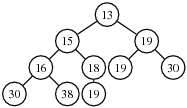Fig. 2 A partially ordered tree.

55.        Explain the notion "heap".

56.        What is an AVL tree?

57.        Draw the AVL tree which result from inserting the keys 52, 04, 09, 35, 43, 17, 22, 11 in an empty tree.

58.        Draw the AVL tree resulting after deleting node 09 from the tree of Fig. 3.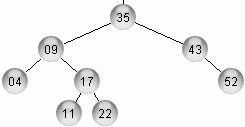Fig. 3. An AVL tree.

59.        Describe the left-right double rotation in an AVL tree.

60.        Draw the AVL tree resulting after deleting node 35 from the tree of Fig. 4.Fig. 4. Another AVL tree.

61.        What can you say about the running time for AVL tree operations?

62.        What is a 2-3 tree?

63.        Show the 2-3 tree which results after inserting the key 13 in the tree of Fig. 5.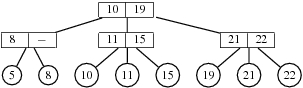Fig. 5. A 2-3 tree.

64.        Show the 2-3 tree which results after deleting the key 8 in the tree of Fig. 5.

65.        What is a 2-3-4 tree?

66.        What were the disjoint sets with union and find designed for?

67.        Define the operations of the union-find set ADT.

68.        Draw a sketch showing a lists implementation for the union-find set ADT with sets: 1: {1, 4, 7}; 2: {2, 3, 6, 9};  8:{8, 11, 10, 12}.

69.        Draw a sketch showing a tree forest implementation for the union-find set ADT with sets: 1: {1, 4, 7}; 2: {2, 3, 6, 9};  8:{8, 11, 10, 12}.

70.        How can one speed up union-find ADT operations?

From this point: Review questions for Final

1. Give an adjacency matrix representation for the graph of Fig. 6.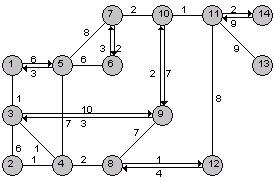Fig.6 A graph.

1. Give an adjacency list representation for the graph of Fig 6.
2. How do adjacency list/matrix representations for graphs compare and when should one ore another be used?
3. Apply Dijkstra’s algorithm for the graph of Fig. 6 starting at node 1.
4. Apply Prim’s algorithm to the graph of Fig 7.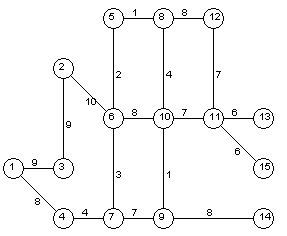Fig 7. Another graph.

1. Apply Kruskal’s algorithm to the graph of Fig. 8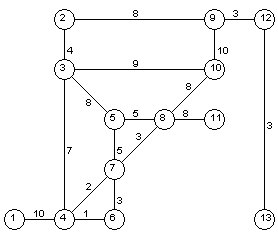Fig. 8 Yet another graph.

1. Apply Bellman-Ford algorithm to the graph of Fig. 9.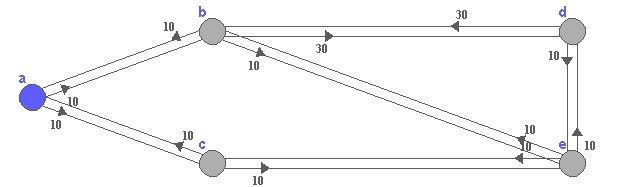Fig.9. A digraph

1. Apply Floyd’s algorithm to the graph of Fig 10.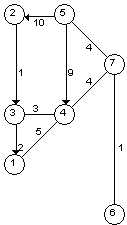Fig.10. Another graph.

1. Use breadth-first search to determine the articulation points of the graph of Fig. 10.
2. Use depth-first search to determine the articulation points of the graph Fig. 10.
3. Determine the transitive closure of the graph of Fig. 10.
4. Apply topological sort to the dag of Fig. 11.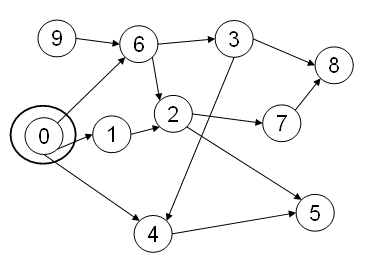Fig. 11. A Directed Acyclic Graph.
1. Develop a short algorithm for finding cycles in a graph.
2. Consider the following MAXMIN algorithm. How many comparisons does it use? Is it likely to be faster or slower than the divide-and-conquer algorithmin practice?
procedure maxmin2(S)
comment computes maximum and minimum of S[1..n] in max and min resp.
1. if n is odd then max:=S[n]; min:=S[n]
2. else max:=−∞; min:=
3. for i := 1 to n/2 do
4.   if S[2i 1] S[2i]
5.         then small:=S[2i 1]; large:=S[2i]
6.         else small:=S[2i]; large:=S[2i 1]
7.   if small < min then min:=small
8.   if large > max then min:=small
1. A sequence of numbers < a1, a2, a3,…, an > is oscillating if ai < ai+1 for every odd index i  and ai > ai+1 for every even index i. Describe and analyze an efficient algorithm to compute the longest oscillating subsequence in a sequence of n integers.
2. Find the following spanning trees for the weighted graph shown in Figure 12, below.
(a) A breadth-first spanning tree rooted at s.
(b) A depth-first spanning tree rooted at s.
(c) A shortest-path tree rooted at s.
(d) A minimum spanning tree.
You do not need to justify your answers; just clearly indicate the edges of each spanning tree. Yes, one of the edges has negative weight.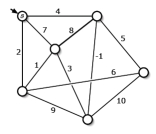Fig. 12. A weighted graph.

1. Describe and analyze an algorithm to compute the size of the largest connected component of black pixels in an n × n bitmap B[1..n; 1..n]. For example, given the bitmap in Figure 13 (below) as input, your algorithm should return the number 9, because the largest conected black component (marked with white dots on the right) contains nine pixels.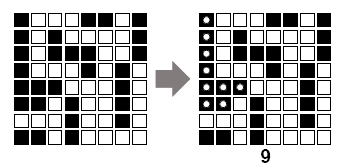Figure 13. Bitmap example.
1. Describe and analyze an algorithm that determines whether a given graph is a tree, where the graph is represented by an adjacency list.
1. Solve the recurrence T(n) = 5T(n/17) + O(n4/3
2. Solve the recurrence T(n) = 1/n + T(n − 1), where T(0) = 0.
3. Suppose you are given an array of n numbers, sorted in increasing order.
(a)  Describe an O(n)-time algorithm for the following problem: Find two numbers from the list that add up to zero, or report that there is no such pair. In other words, find two numbers a and b such that a + b = 0.
(b)  Describe an O(n2)-time algorithm for the following problem: Find three numbers from the list that add up to zero, or report that there is no such triple. In other words, find three numbers a, b, and c, such that a+b+c = 0. [Hint: Use something similar to part (a) as a subroutine.]
1.  Sketch the selection sort algorithm and show how it works on the array containing the keys 82, 31, -13, 45,  99,  -1, -7, 22.
2.  Sketch the insertion sort algorithm and show how it works on the array containing the keys 82, 31, -13, 45,  99,  -1, -7, 22.
3.  Sketch the merge sort algorithm and show how it works on the array containing the keys 82, 31, -13, 45,  99,  -1, -7, 22.
4.  Sketch the quick sort algorithm and show how it works on the array containing the keys 82, 31, -13, 45,  99,  -1, -7, 22.
5.  Sketch the radix sort algorithm and show how it works on the array containing the keys 82, 31, -13, 45,  99,  -1, -7, 22.
6.  Sketch the counting sort algorithm and show how it works on the array containing the keys 2, 1, 5, 5,  9,  3, 7, 2.
7.  Sketch the bucket sort algorithm and show how it works on the array containing the keys 0.82, 0.31, 0.13, 0.45,  0.99,  0.1, 0.7, 0.22.
8.  What are the criteria used to evaluate the running time of an internal sorting algorithm?
9.  How do we select a sort algorithm?
10.  What strategies ca be involved for selecting a new E-vertex with branch and bound?
11.  Compare the backtracking and branch and bound search strategies.
12.  Describe the local search strategy.
13.  Apply the local search strategy to the problem of finding the MST of the graph shown in Figure 14, below: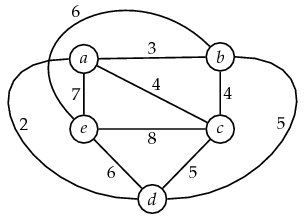Fig. 14. Another weighted graph.
1. Describe the Divide and Conquer method for algorithm design.
2. Describe the steps taken when applying dynamic programming strategy when developing an algorithm.
3. Suppose we have 220 128-bit elements that we would like to sort. What would be the efficiency of sorting these using quicksort? What would be the efficiency of sorting these as radix-216 numbers using radix sort? Which approach would be better? Suppose we have 210 128-bit elements rather than 220 elements. How do quicksort and radix sort compare in this case?
4. In a sorted set, the successor of some node x is the next largest node after x. For example, in a sorted set containing the keys 24, 39, 41, 55, 87, 92, the successor of 41 is 55. How do we find the successor of an element x using binary search? What is the runtime complexity of this operation?
5. Suppose we model an internet using a graph and we determine that the graph contains an articulation point. What are the implications of this?
6. Consider a graph that models a structure of airways, highways in the sky on which airplanes are often required to fly. The structure consists of two types of elements: navigational facilities, called navaids for short, and airways that connect navaids, which are typically within a hundred miles of each other. Airways may be bidirectional or one-way. At certain times some airways are not available for use. Suppose during one of these times we would like to determine whether we can still reach a particular destination. How can we determine this? What is the runtime complexity of solving this problem?
7. Suppose we would like to use a computer to model states in a system. For example, imagine the various states of a traffic-light system at an intersection and the decisions the system has to make. How can we use a graph to model this?
8. The transpose of a directed graph is a graph with the direction of its edges reversed. Formally, for a directed graph G = (V, E ), its transpose is indicated as GT. How could we form the transpose of a graph assuming an adjacency-list representation? What is the runtime complexity of this?
9. The following recursive definition has an error. What is it, and how can we fix it? For a positive integer n, the definition, in its proper form, is common in formally computing the running time of divide-and-conquer algorithms, such as merge sort. Merge sort divides a set of data in half, then divides the halves in half, and continues this way until each division contains a single element. Then, during the unwinding phase, the divisions are merged to produce a final sorted set.
1. Analyze the asymptotic time complexity T(n) for the following two divide-and-conquer algorithm. You may assume that n is a power of 2.
int foo(A)
{
n = A.length;
if (n==1)
{
return A;
}
int half = (int) n/2
int[] A1 = new int[half];
int[] A2 = new int[n-half];
for (int i=0; i <= half-1; i++)
{
for (int j=0; j <= 1; j++)
{
if (j==0) A1[i] = A[2i];
else A2[i] = A[2i+1];
}
}
A2[n-half-1] = A[n-1];
b1 = foo(A1);
b2 = foo(A2);
if (b1>b2) return b1;
else return b2;
}
1. Consider a game tree in which there are six marbles, and players 1 and 2 take turns picking from one to three marbles. The player who takes the last marble loses the game.

1. Give an algorithm that takes an array of n numbers (n is even) and finds the maximum one and the minimum one in 3(n/2)-2 comparisons.
2. Suppose you have n integers in the range from 0 to n3-1. Explain how to sort them in O(n) time.
3. Develop an algorithm to solve the basic Tower of Hanoi problem, i.e., 3 towers, N disks, and the given rules.
4. How could you make an algorithm for finding the longest path in a graph with only negative weights?
5. Suppose there are three alternatives for dividing a problem of size n into subproblems of smaller size: if you solve 3 subproblems of size n/2 , then the cost for combining the solutions of the subproblems to obtain a solution for the original problem is Theta(n2 sqrt(n)); if you solve 4 subproblems of size n/2, then the cost for combining the solutions is Theta(n2 ); if you solve 5 subproblems of size n/2 , then the cost for combining the solutions is Theta(n log n). Which alternative do you prefer and why?
6. A palindrome is a word w1w2 . . .wk whose reverse wkwk−1 . . .w1 is the same string, e.g. “abbabba”. Consider a string A = a1a2 . . . an. A partitioning of the string is a palindrome partitioning if every substring of the partition is a palindrome. For example, “aba|b|bbabb|a|b|aba” is a palindrome partitioning of “ababbbabbababa”. Design a dynamic programming algorithm to determine the coarsest (i.e. fewest cuts) palindrome partition of A.
7. Consider a directed graph G = (V,E) where each edge is labeled with a character from an alphabet Sigma, and we designate a special vertex s as the start vertex, and another f as the final vertex. We say that G accepts a string A = a1a2 . . . an if there is a path from s to f of n edges whose labels spell the sequence A. Design an O((|V | + |E|)n) dynamic programming algorithm to determine whether or not A is accepted by G.
8. Give the pseudocode for a greedy algorithm that solves the following optimization problem. Justify briefly why your algorithm finds th optimum solution. What is the asymptotic running time of your algorithm in terms of n?
9. 1,…Sn on E60 highway from Cluj-Napoca to Oradea. On a full tank of gas, your car goes for D miles. Gas station S1 is in Cluj-Napoca, and each gas station Si for 2 <= i <= n, is at di < D kilometers after the previous gas station Si-1, and gas station Sn is in Oradea. What is the minimum number of gas stops you must make when driving from Cluj-Napoca to Oradea?
10. Discuss the solution for Travelling salesman problem using branch & bound technique.
11. Apply backtracking technique to solve the following instance of subset sum problem : S={1,3,4,5} and d=11.
12. The configuration of a playground is given as an n by n grid. Each of the elements in the grid has an elevation, expressed as a positive integer number. Someone places a ball at coordinates (i,j) on this playground. The ball then moves to a lower elevation in neighboring locations. List all the possibilities for the ball to move in order to leave the playground. What is the running time of your algorithm?
13. There is a n <= 26 persons, identified by capital letters, each person having at least n/2 friends. One of these persons owns a book, which the rest of the persons wish to read. Find all ways of passing the book to all the friends of the owner, starting at the owner, and reaching each friends exactly once. Finally, the book should be handed back to the owner. What is the running time of your algorithm?
14. Assume we are given a connected, undirected graph G, with n nodes and m edges. Develop an algorithm to set directions for the edges of the graph such a way that from every vertex there should be an even number of outgoing arcs. What is the running time of your algorithm?
15. Develop and algorithm which constructs shedules for round-robin tournaments (for games like tennis, chess, voleyball, soccer, etc.), where the number of players is not a power of 2. Players (at most 30) are identified by numbers. What algorithm design method did you use? What is the running time of your algorithm?
16. On a chess board, there are m obstacles given by their board coordinates. Also, on that board there is a bishop located at coordinates (i,j) (the upper left corner has coordinates (0,0)). Find the path with the minimum number of moves, which obey the rules of chess game, for the bishop to reach a given position (p,q), avoiding the obstacles.
17. Draw the B+Tree which results after insertion of key 70 in the B+tree below.
1. Draw the B+Tree which results after insertion of key 95 in the B+tree below.
1. Draw the B+Tree which results after deletion of key 25 in the B+tree below.
1. What is a multi-way search tree of order m?
2. Use Use the alpha/beta procedure to identify which parts of the tree can be pruned. Clearly identify alpha and beta pruning.
1. An architect has been commissioned to design a new building, called the Data Center. Gary wants his top architectural protégé to design a scale model of the Data Center using precision-cut sticks, but he wants to preclude the model from inadvertently containing any right angles. Gary fabricates a set of n sticks, labeled 1,2,...,n, where stick i has length xi. Before giving the sticks to the protégé, he shows them to you and asks you whether it is possible to create a right triangle using any three of the sticks. Give an efficient algorithm for determining whether there exist three sticks a, b, and c such that the triangle formed from them — having sides of lengths xa, xb, and xc — is a right triangle (that is, xa2 + xb2 = xc2).
2. Argue that any comparison based sorting algorithm can be made to be stable, without affecting the running time by more than a constant factor.
3. Define a linked list with a loop as a linked list in which the tail element points to one of the list’s elements and not to NULL. Assume that you are given a linked list L, and two pointers P1, P2 to the head. Write an algorithm that decides whether the list has a loop without modifying the original list. The algorithm should run in time O(n) and additional memory O(1), where n is the number of elements in the list.
4. Use a min-heap to give an O(n log k)-time algorithm which merges k sorted lists into one sorted list, where n is the total number of elements in all the input lists.
5. Let G = (V,E) be an undirected graph, and s be a node in V . Edge (u, v) 2 E is called a circular edge if the distance from s to u is identical to the distance from s to v. Give a linear algorithm that given s finds all circular edges in graph. Explain what data structures you use, and provide complexity analysis.
6. Give (in pseudocode) an O(|V | + |E|) algorithm that takes as input a directed acyclic graph and two vertices s, t, and returns the number of paths from s to t in G. Your algorithm needs only to count the paths, not list them. Hint: Use topological sort as a part of your solution.
7. Give an algorithm to either output a message “No counterfeit coin”, or identify which of three coins: A, B and C is counterfeit.
8. Construct a divide and conquer algorithm that divides a set S set of coins into three equal subsets, and uses part (a) to solve small (i.e. 3-coin) sets.
1. Suppose you have analysed a file and determined that the frequency of occurrence of certain characters is as follows:

Character         a        b        c        d        e        f

Occurences    15      7        5        8        30      10

a) Construct the Huffman tree for the characters

b) List the codes for each character

c) Use the tree to compress the following strings:

i) faded’                 ii) ‘abed’

iii)‘feed’